Equation of circle passing through 3 given points

# P(2,1), Q(0,5), R(-1,2)

## 1

### Substitute points in the general form of circle and solve coefficients

Let the circle be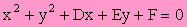(2,1) :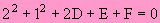(0,5) :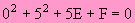(-1,2) :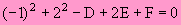Substitution of P, Q, R.

2D + E + F = -5 ….. (1)

5E + F = -25 ………(2)

D – 2E – F = 5 …….(3)

Simplification

(1) + (3),   3D – E = 0 ……. (4)

(2) + (3),   D + 3E = -20 ….. (5)

From (4),   E = 3D …………(6)

Subst. (6) in (5),  D + 9D = -20

\ D = -2 ………………. (7)

Subst. (7) in (6),  E = -6 …….. (8)

Subst. (7), (8) in (1),  F = -5

Solve the equations.

Eliminating F and get a simultaneous equation

in D and E.

Solve D and E

and finally get F.

The equation of the circle is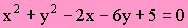Substitute D, E, F

in the General Form.

# P(2,1), Q(0,5), R(-1,2)

## 2

### Use Centre and Radius Form of the circle.

Let the center and radius of the circle be C(a,b) and r.

|PC| = |QC| = |RC|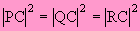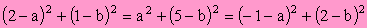The distance from center to the given 3 points are equal.

5 - 4a - 2b = 25 - 10b = 5 + 2a - 4b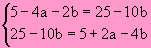Simplification.

Note that there are no “square” terms.

Get a simultaneous equation in a and b.

(2) – (1),  5b = 15

\ b = 3 ….. (3)

Subst. (3) in (1),   a = 1

\ C = (3, 1)

Solve a and b.

The center of the circle can be found.

r = |PC|The equation of the circle is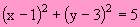Use the Center- radius form to get the equation of circle.

# P(2,1), Q(0,5), R(-1,2)

## 3

### The perpendicular bisectors of two chords meet at the centre.

Let L1 and L2 be the perpendicular bisectors of PQ and QR respectively.

Mid-point of PQ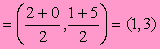Mid-point of QR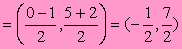Gradient of PQ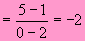Gradient of QR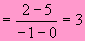Since L1 ^ PQ,  L2 ^ QR,

Gradient of L1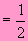Gradient of L2 =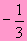L1 :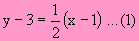L2 :Find the mid-points of two chords.

Find the gradient of two chords.

Product of gradients of perpendicular lines = -1

Find the equations of perpendicular bisectors using gradient-point form.

(1) – (2),\ x = 1 …. (3)

Subst. (3) in (1), \ y = 3

\ C = (1,3)

Solve for the center.

r = |PC|The equation of the circle isUse the Center- radius Form to get the equation of circle.

We use another set of three points to work for the other methods below.

# P(-6,5), Q(-3,-4), R(2,1)

## 4

### Converse of Angle in semi-circle.

Gradient of PQ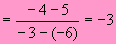Gradient of PR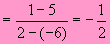Let L1 ^ PQ and  L2 ^ PR.

Let L1 and L2 meet at S.

Gradient of L1 =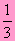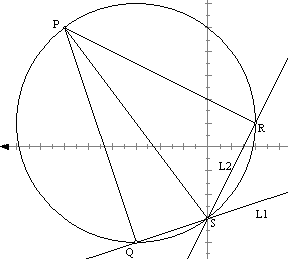Product of gradients of perpendicular lines = -1

Equation of L1:Equation of L2: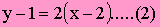(1) – (2),     \ x = 0 ….. (3)

Subst. (3) in (1),  y = -3.

\ S = (0, -3)

Use Point-gradient form to find equations of L1 and L2.

Solve the equations, the point S can be found.

P = (-6, 5)   S = (0, -3)

PS is the diameter of the circle.

The equation of the circle:

(x + 6)(x - 0) + (y – 5)(y + 3) = 0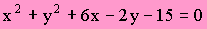Apply the theorem of Converse of Angle in Semi-circle we can see that P, Q, R, S are concyclic with PS as diameter.

Use diameter form to find equation of circle.

# P(-6,5), Q(-3,-4), R(2,1)

## 5

### System of circles.

Equation of PQ :L :  3x + y + 13 = 0

Circle with PQ as diameter:

C : (x +6)(x+3) + (y –5)(y + 4) = 0Two points form.

Write PQ in general form.

Use the diameter form to find the circle with PQ as diameter.

The system of circle passing through the intersections of the circle C and the line L can be given by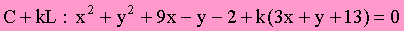This system of circles must pass through points P and Q.

We like to find one of the circles in this system which passes through the point R (2,1).

Subst. R(2,1) in C + kL we have,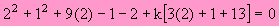\ k = -1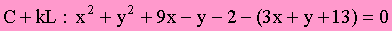The required circle is :Find k.

The system of circles passes through P, Q.

The circle also passes through R.

# P(-6,5), Q(-3,-4), R(2,1)

## 6

### Angles in same segment .

Gradient of PQGradient of PR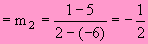Let a be the angle  QPR.…..(1)

You may investigate the case for which the absolute value is added: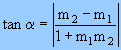Let S(x, y) be any point on the circle.

Gradient of SQ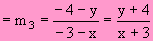Gradient of SR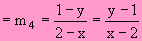Let b be the angle QSR.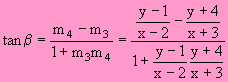….. (2)

We do not include the absolute value in the formula.

The order of m is therefore important.

Since a = b, therefore tan a = tan b

Equate (1) and (2), we get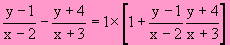Simplify, we get the equation of circle:Use the Angles in the same segment.

If we consider the absolute value previously, we have to add ± sign in (1) and (2).

Use Opposite angles of cyclic quad. supplementary,

we get    a = 180°-b

tan a = - tan b

the negative sign is taken care.

# P(-6,5), Q(-3,-4), R(2,1)

## 7

### Determinant method

The required circle is of the form: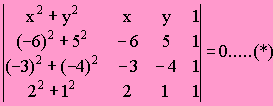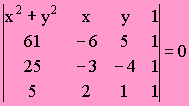Reasons:

(1) The equation is of second degree and is a conic.

(2) Coefficients of x^2 = y^2

and no xy- term, it is a circle.

(3) Subst. P(-6, 5) in (*),

we get R1 and R2 are equal and therefore P satisfies (*).

Similarly for Q and R. Therefore the circle passes through P, Q, R.

Using Laplace expansion we get: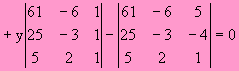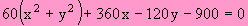Simplify, we get the equation of circle:Although (*) is very simple and compact, its evaluation is complicate. You should know how to break down a higher order determinant.